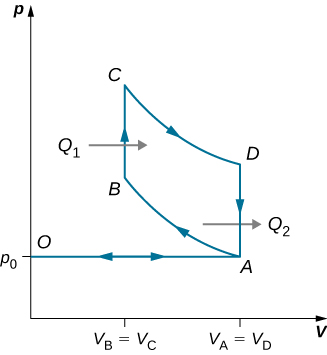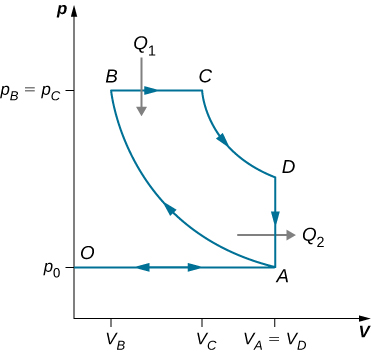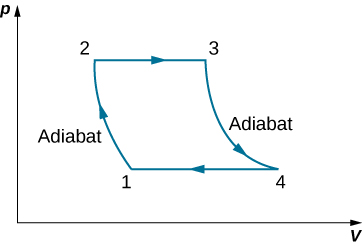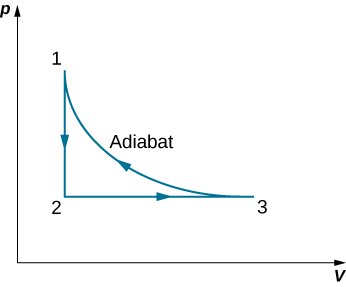# 4.7 Entropy on a microscopic scale  (Page 4/10)

 Page 7 / 10

A 0.50-kg piece of aluminum at $250\phantom{\rule{0.2em}{0ex}}\text{°}\text{C}$ is dropped into 1.0 kg of water at $20\phantom{\rule{0.2em}{0ex}}\text{°}\text{C}$ . After equilibrium is reached, what is the net entropy change of the system?

82 J/K

Suppose 20 g of ice at $0\phantom{\rule{0.2em}{0ex}}\text{°}\text{C}$ is added to 300 g of water at $60\phantom{\rule{0.2em}{0ex}}\text{°}\text{C}$ . What is the total change in entropy of the mixture after it reaches thermal equilibrium?

A heat engine operates between two temperatures such that the working substance of the engine absorbs 5000 J of heat from the high-temperature bath and rejects 3000 J to the low-temperature bath. The rest of the energy is converted into mechanical energy of the turbine. Find (a) the amount of work produced by the engine and (b) the efficiency of the engine.

a. 2000 J; b. $40\text{%}$

A thermal engine produces 4 MJ of electrical energy while operating between two thermal baths of different temperatures. The working substance of the engine rejects 5 MJ of heat to the cold temperature bath. What is the efficiency of the engine?

A coal power plant consumes 100,000 kg of coal per hour and produces 500 MW of power. If the heat of combustion of coal is 30 MJ/kg, what is the efficiency of the power plant?

$60\text{%}$

A Carnot engine operates in a Carnot cycle between a heat source at $550\phantom{\rule{0.2em}{0ex}}\text{°}\text{C}$ and a heat sink at $20\phantom{\rule{0.2em}{0ex}}\text{°}\text{C}.$ Find the efficiency of the Carnot engine.

A Carnot engine working between two heat baths of temperatures 600 K and 273 K completes each cycle in 5 sec. In each cycle, the engine absorbs 10 kJ of heat. Find the power of the engine.

$64.4\text{%}$

A Carnot cycle working between $100\phantom{\rule{0.2em}{0ex}}\text{°}\text{C}$ and $30\phantom{\rule{0.2em}{0ex}}\text{°}\text{C}$ is used to drive a refrigerator between $-10\phantom{\rule{0.2em}{0ex}}\text{°}\text{C}$ and $30\phantom{\rule{0.2em}{0ex}}\text{°}\text{C}.$ How much energy must the Carnot engine produce per second so that the refrigerator is able to discard 10 J of energy per second?

## Challenge problems

(a) An infinitesimal amount of heat is added reversibly to a system. By combining the first and second laws, show that $dU=TdS-dW$ . (b) When heat is added to an ideal gas, its temperature and volume change from ${T}_{1}\phantom{\rule{0.2em}{0ex}}\text{and}\phantom{\rule{0.2em}{0ex}}{V}_{1}\phantom{\rule{0.2em}{0ex}}\text{to}\phantom{\rule{0.2em}{0ex}}{T}_{2}\phantom{\rule{0.2em}{0ex}}\text{and}\phantom{\rule{0.2em}{0ex}}{V}_{2}$ . Show that the entropy change of n moles of the gas is given by

$\text{Δ}S=n{C}_{v}\phantom{\rule{0.2em}{0ex}}\text{ln}\frac{{T}_{2}}{{T}_{1}}+nR\phantom{\rule{0.2em}{0ex}}\text{ln}\frac{{V}_{2}}{{V}_{1}}$ .

derive

Using the result of the preceding problem, show that for an ideal gas undergoing an adiabatic process, $T{V}^{\text{γ}-1}$ is constant.

With the help of the two preceding problems, show that $\text{Δ}S$ between states 1 and 2 of n moles an ideal gas is given by

$\text{Δ}S=n{C}_{p}\phantom{\rule{0.2em}{0ex}}\text{ln}\frac{{T}_{2}}{{T}_{1}}-nR\phantom{\rule{0.2em}{0ex}}\text{ln}\frac{{p}_{2}}{{p}_{1}}$ .

derive

A cylinder contains 500 g of helium at 120 atm and $20\phantom{\rule{0.2em}{0ex}}\text{°}\text{C}$ . The valve is leaky, and all the gas slowly escapes isothermally into the atmosphere. Use the results of the preceding problem to determine the resulting change in entropy of the universe.

A diatomic ideal gas is brought from an initial equilibrium state at ${p}_{1}=0.50\phantom{\rule{0.2em}{0ex}}\text{atm}$ and ${T}_{1}=300\phantom{\rule{0.2em}{0ex}}\text{K}$ to a final stage with ${p}_{2}=0.20\phantom{\rule{0.2em}{0ex}}\text{atm}$ and ${T}_{1}=500\phantom{\rule{0.2em}{0ex}}\text{K}.$ Use the results of the previous problem to determine the entropy change per mole of the gas.

18 J/K

The gasoline internal combustion engine operates in a cycle consisting of six parts. Four of these parts involve, among other things, friction, heat exchange through finite temperature differences, and accelerations of the piston; it is irreversible. Nevertheless, it is represented by the ideal reversible Otto cycle , which is illustrated below. The working substance of the cycle is assumed to be air. The six steps of the Otto cycle are as follows:

1. Isobaric intake stroke ( OA ). A mixture of gasoline and air is drawn into the combustion chamber at atmospheric pressure ${p}_{0}$ as the piston expands, increasing the volume of the cylinder from zero to ${V}_{A}$ .
2. Adiabatic compression stroke ( AB ). The temperature of the mixture rises as the piston compresses it adiabatically from a volume ${V}_{\text{A}}\phantom{\rule{0.2em}{0ex}}\text{to}\phantom{\rule{0.2em}{0ex}}{V}_{\text{B}}$ .
3. Ignition at constant volume ( BC ). The mixture is ignited by a spark. The combustion happens so fast that there is essentially no motion of the piston. During this process, the added heat ${Q}_{1}$ causes the pressure to increase from ${p}_{B}\phantom{\rule{0.2em}{0ex}}\text{to}\phantom{\rule{0.2em}{0ex}}{p}_{C}$ at the constant volume ${V}_{\text{B}}\left(={V}_{\text{C}}\right)$ .
4. Adiabatic expansion ( CD ). The heated mixture of gasoline and air expands against the piston, increasing the volume from ${V}_{C}\phantom{\rule{0.2em}{0ex}}\text{to}\phantom{\rule{0.2em}{0ex}}{V}_{D}$ . This is called the power stroke , as it is the part of the cycle that delivers most of the power to the crankshaft.
5. Constant-volume exhaust ( DA ). When the exhaust valve opens, some of the combustion products escape. There is almost no movement of the piston during this part of the cycle, so the volume remains constant at ${V}_{A}\left(={V}_{D}\right)$ . Most of the available energy is lost here, as represented by the heat exhaust ${Q}_{2}$ .
6. Isobaric compression ( AO ). The exhaust valve remains open, and the compression from ${V}_{A}$ to zero drives out the remaining combustion products.

(a) Using ( i ) $e=W\text{/}{Q}_{1}$ ; ( ii ) $W={Q}_{1}-{Q}_{2}$ ; and ( iii ) ${Q}_{1}=n{C}_{v}\left({T}_{C}-{T}_{B}\right)$ , ${Q}_{2}=n{C}_{v}\left({T}_{D}-{T}_{A}\right)$ , show that

$e=1-\frac{{T}_{D}-{T}_{A}}{{T}_{C}-{T}_{B}}$ .

(b) Use the fact that steps (ii) and (iv) are adiabatic to show that

$e=1-\frac{1}{{r}^{\gamma -1}}$ ,

where $r={V}_{A}\text{/}{V}_{B}$ . The quantity r is called the compression ratio of the engine.

(c) In practice, r is kept less than around 7. For larger values, the gasoline-air mixture is compressed to temperatures so high that it explodes before the finely timed spark is delivered. This preignition causes engine knock and loss of power. Show that for $r=6$ and $\gamma =1.4$ (the value for air), $e=0.51$ , or an efficiency of $51\text{%}.$ Because of the many irreversible processes, an actual internal combustion engine has an efficiency much less than this ideal value. A typical efficiency for a tuned engine is about $25\text{%}\phantom{\rule{0.2em}{0ex}}\text{to}\phantom{\rule{0.2em}{0ex}}30\text{%}$ .An ideal diesel cycle is shown below. This cycle consists of five strokes. In this case, only air is drawn into the chamber during the intake stroke OA . The air is then compressed adiabatically from state A to state B , raising its temperature high enough so that when fuel is added during the power stroke BC , it ignites. After ignition ends at C , there is a further adiabatic power stroke CD . Finally, there is an exhaust at constant volume as the pressure drops from ${p}_{D}$ to ${p}_{A}$ , followed by a further exhaust when the piston compresses the chamber volume to zero.

(a) Use $W={Q}_{1}-{Q}_{2}$ , ${Q}_{1}=n{C}_{p}\left({T}_{C}-{T}_{B}\right)$ , and ${Q}_{2}=n{C}_{v}\left({T}_{D}-{T}_{A}\right)$ to show that $e=\frac{W}{{Q}_{1}}=1-\frac{{T}_{D}-{T}_{A}}{\gamma \left({T}_{C}-{T}_{B}\right)}$ .

(b) Use the fact that $A\to B$ and $C\to D$ are adiabatic to show that

$e=1-\frac{1}{\gamma }\phantom{\rule{0.2em}{0ex}}\frac{{\left(\frac{{V}_{C}}{{V}_{D}}\right)}^{\gamma }-{\left(\frac{{V}_{B}}{{V}_{A}}\right)}^{\gamma }}{\left(\frac{{V}_{C}}{{V}_{D}}\right)-\left(\frac{{V}_{B}}{{V}_{A}}\right)}$ .

(c) Since there is no preignition (remember, the chamber does not contain any fuel during the compression), the compression ratio can be larger than that for a gasoline engine. Typically, ${V}_{A}\text{/}{V}_{B}=15\phantom{\rule{0.2em}{0ex}}\text{and}\phantom{\rule{0.2em}{0ex}}{V}_{D}\text{/}{V}_{C}=5$ . For these values and $\gamma =1.4,$ show that $\epsilon =0.56$ , or an efficiency of $56\text{%}$ . Diesel engines actually operate at an efficiency of about $30\text{%}\phantom{\rule{0.2em}{0ex}}\text{to}\phantom{\rule{0.2em}{0ex}}35\text{%}$ compared with $25\text{%}\phantom{\rule{0.2em}{0ex}}\text{to}\phantom{\rule{0.2em}{0ex}}30\text{%}$ for gasoline engines.proof

Consider an ideal gas Joule cycle, also called the Brayton cycle, shown below. Find the formula for efficiency of the engine using this cycle in terms of ${P}_{1}$ , ${P}_{2}$ , and $\gamma$ .Derive a formula for the coefficient of performance of a refrigerator using an ideal gas as a working substance operating in the cycle shown below in terms of the properties of the three states labeled 1, 2, and 3.${K}_{\text{R}}=\frac{3\left({p}_{1}-{p}_{2}\right){V}_{1}}{5{p}_{2}{V}_{3}-3{p}_{1}{V}_{1}-{p}_{2}{V}_{1}}$

Two moles of nitrogen gas, with $\gamma =7\text{/}5$ for ideal diatomic gases, occupies a volume of ${10}^{-2}{\text{m}}^{3}$ in an insulated cylinder at temperature 300 K. The gas is adiabatically and reversibly compressed to a volume of 5 L. The piston of the cylinder is locked in its place, and the insulation around the cylinder is removed. The heat-conducting cylinder is then placed in a 300-K bath. Heat from the compressed gas leaves the gas, and the temperature of the gas becomes 300 K again. The gas is then slowly expanded at the fixed temperature 300 K until the volume of the gas becomes ${10}^{-2}{\text{m}}^{3}$ , thus making a complete cycle for the gas. For the entire cycle, calculate (a) the work done by the gas, (b) the heat into or out of the gas, (c) the change in the internal energy of the gas, and (d) the change in entropy of the gas.

A Carnot refrigerator, working between $0\phantom{\rule{0.2em}{0ex}}\text{°}\text{C}$ and $30\phantom{\rule{0.2em}{0ex}}\text{°}\text{C}$ is used to cool a bucket of water containing ${10}^{-2}\phantom{\rule{0.2em}{0ex}}{\text{m}}^{3}$ of water at $30\phantom{\rule{0.2em}{0ex}}\text{°}\text{C}$ to $5\phantom{\rule{0.2em}{0ex}}\text{°}\text{C}$ in 2 hours. Find the total amount of work needed.

$W=110,000\phantom{\rule{0.2em}{0ex}}\text{J}$

Why does the lines of force not touch each other 🇲🇲
what is unit
Please canu get more questions on electric field and electric flux please
Gbemisola
is electric field directly proportional to the squared of a distance
No electric field is inversely proportional to the squared distance between the charges
Gbemisola
The bullet 2.00cm long is fired at 420/s and passes straight through a 10.0 cm thick board existing at 280 m/s.What is the average acceleration of the bullet through the board?
FAUSTINA
an unstretched spring is 12cm long .A load of 5N stretched it to 15cm .how long will it be under a load of 15N?
Morapeli
hi
Africa
hi
Benjamin
Benjamin how are u are u a freshman in the university
Africa
like 100 level
Africa
yes sir
Benjamin
l need a physics tutor
Benjamin
I think the best tutor is God and organic tutor in YouTube that guy is good
Africa
me too 100level
Africa
wow nice
Benjamin
from Nigeria and u
Africa
I am from Nigeria and u wow nice that something I use to always say
Africa
am from Ghana
Benjamin
ok
Africa
studying what
Africa
Compare the electric flux through the surface of a cube of side length a that has a charge q at its center to the flux through a spherical surface of radius a with a charge q at its center.
please I want to know how to solve increase in length
Ujah
Why a charged capacitor has potential difference but not emf
what is the dimension symbol of temperature?
what is the dimension symbol of temperature?
Keren
what's the meaning of enthalpy in terms of latent heat, internal energy, phase change
Enthalpy is the degree ofdisorderlinessof a substance
Gbemisola
how to convert Kelvin to centigrade
what is the s, p, d, f in this table
Sangeetha
s, p, d, f in this table
Sangeetha
what kind of table this
Vengata
Periodic table
Gbemisola
what are waves
In physics, mathematics, and related fields, a wave is a propagating dynamic disturbance (change from equilibrium) of one or more quantities
Discuss how would orient a planar surface of area A in a uniform electric field of magnitude E0 to obtain (a) the maximum flux and (b) the minimum flux through the area.
I'm just doing the first 3 with this message. but thankyou for the time your obviously intending to support us with. viva la accumulation
Marcel
Find the net capacitance of the combination of series and parallel capacitors shown belo
what is ohm?
calculate ideal gas pressure of 0.300mol,v=2L T=40°c
what is principle of superposition

#### Get Jobilize Job Search Mobile App in your pocket Now!By Royalle MooreBy Frank LevyByBy Stephen VoronBy OpenStaxBy OpenStaxBy Yasser IbrahimBy Melinda SalzerBy OpenStaxBy Caitlyn Gobble